# Tkinter的Treeview组件增加，删除，修改，设置等操作

### 在第1列图标栏为一行记录设一个图标

`tree1.insert(sf1, END, text='广州市',values=('海珠区','阅江中路380号'))`

`tree1.insert(sf1, END, text='广州市',values=('海珠区','阅江中路380号') ,image=img1)`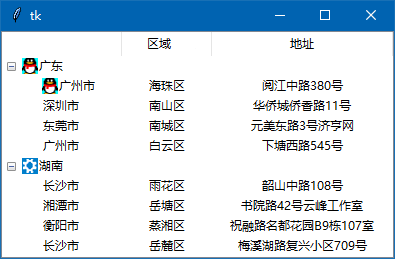可以看到在“广东”节点下的“广州市”也可以出现图标。

### 为一列的标题栏设一个图标

`tree1.heading('qy', text='区域',image=img1)`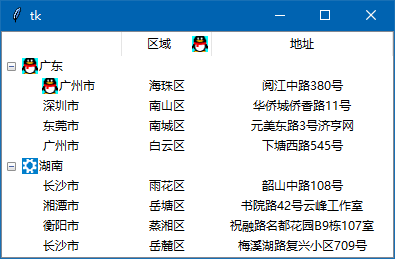### 获取一行的文本，获取一行中某一项的文本：

insert(parent, index, iid=None, **kw)

parent : 对于有树栏的Treeview，parent是父节点，对于只是列表栏的Treeview，parent一般为空。

index ：插入位置。可以是END或’end’ ，也可以是数字的，如果你想新插入的item(记录)成为第某节点的第一个，index就设为0，以此类推。

iid : 每一行记录（item）的标识符，这个参数，我上面没有讲解，等下，我会专门讲解。

**kw ：设置插入的记录（item）所支持属性，我前面基本都讲解了，只有tags 这个标签参数没有讲解，下面我也会专门讲解。丶丌皛

 参数 说明 text 树栏，第1列显示的文本 values 第2列以后显示的文本，元组元素为各列显示的文本 image 每行显示在第1列的图标 open 在树栏中，节点是否展开，True展开，False折叠 tags 为插入的item设置tags标签

```sf1 = tree1.insert('', END, text='广东',image=img1,open=True)
sf2 = tree1.insert('', END, text='湖南',image=img2,open=True)
# 在根节点‘’下添加2个子节点：广东，湖南
print(sf1) # 输出节点sf1的iid值
print(sf2) # 输出节点sf2的iid值```

I001

I002

I001，I002 是系统自动为我们设定的一个iid值，如果我们要自定义iid值，在insert() 方法加上iid参数即可，如：

```sf1 = tree1.insert('', END, text='广东',iid='aaa',image=img1,open=True)
sf2 = tree1.insert('', END, text='湖南',iid='bbb',image=img2,open=True)
# 在根节点‘’下添加2个子节点：广东，湖南
print(sf1) # 输出节点sf1的iid值
print(sf2) # 输出节点sf2的iid值```

aaa

bbb

item( iid )  当参数iid可以确定，返回值就是item的各参数值，是键值对的字典。

item(iid, option=’text’) #  输出text的值

item(iid, option=’values’) # 输出values的值

1.  <<TreeviewSelect>>  选择行发生了变化触发事件

2.  <<TreeviewOpen>>   节点展开时触发事件

3.  <<TreeviewClose>>  节点折叠时触发事件

```from tkinter import *
from tkinter.ttk import *

root = Tk()

img1=PhotoImage(file='16-1.png')
img2=PhotoImage(file='16-2.png')

tree1 = Treeview(root, columns=('qy', 'dz'))
# 创建树表格组件，栏目有3个：#0, qy, dz

tree1.column('#0', width=120, anchor=CENTER)
tree1.column('qy', width=90, anchor=CENTER)
tree1.column('dz', width=180, anchor=CENTER)
# 定义3个栏目的宽度，对齐方法，宽度是否窗体变化

# 定义3个栏目的表头文字

sf1 = tree1.insert('', END, text='广东',image=img1,open=True)
sf2 = tree1.insert('', END, text='湖南',image=img2,open=True)
# 在根节点‘’下添加2个子节点：广东，湖南
print(sf1)

tree1.insert(sf1, END, text='广州市',values=('海珠区','阅江中路380号'))
tree1.insert(sf1, END, text='深圳市',values=('南山区','华侨城侨香路11号'))
tree1.insert(sf1, END, text='东莞市',values=('南城区','元美东路3号济亨网'))
# 在广州（sf1）节点下，插入3条记录：#0栏 = text,其它栏 = values()

tree1.insert(sf2, END, text='长沙市',values=('雨花区','韶山中路108号'))
tree1.insert(sf2, END, text='湘潭市',values=('岳塘区','书院路42号云峰工作室'))
tree1.insert(sf2, END, text='衡阳市',values=('蒸湘区','祝融路名都花园B9栋107室'))
# 在湖南（sf2）节点下，插入3条记录：#0栏 = text,其它栏 = values()

tree1.insert(sf2, END, text='长沙市',values=('岳麓区','梅溪湖路复兴小区709号'))
tree1.insert(sf1, END, text='广州市',values=('白云区','下塘西路545号'))
# 以同样方法插入2条记录，它们会根据父节点找到自己的位置

tree1.pack(fill=BOTH,expand=True)

# 以下为新加代码
def selectjob(event):
print('----------')
print('iid=',tree1.selection()) # 输入选中的行的iid值
print(tree1.item(tree1.selection())) # 输出选中行的各参数值的键值对字典
print('text=',tree1.item(tree1.selection(),option='text')) # 输出某个参数的值

def openjob(event):
print('============')
print(tree1.selection(),'节点展开') # 某iid值的节点被展开
def closejob(event):
print('============')
print(tree1.selection(),'节点折叠') # 某iid值的节点被折叠

tree1.bind("<<TreeviewSelect>>",selectjob) # 某行被选中事件
tree1.bind("<<TreeviewOpen>>",openjob) # 某节点被展开
tree1.bind("<<TreeviewClose>>",closejob) # 某节点被折叠

root.mainloop()```

### 修改一行的文本，修改一行中某一项的文本

1.  item()方法

语法：item(iid, text=文本, values=元组, image=图像对象)

2. set()方法

语法：set(iid，column=列标识符, value=文本)

注：这种方法不能设置第1列（#0）的文本信息

```from tkinter import *
from tkinter.ttk import *

root = Tk() # 源码来自wb98.com

img1=PhotoImage(file='16-1.png')
img2=PhotoImage(file='16-2.png')

tree1 = Treeview(root, columns=('qy', 'dz'))
# 创建树表格组件，栏目有3个：#0, qy, dz

tree1.column('#0', width=120, anchor=CENTER)
tree1.column('qy', width=90, anchor=CENTER)
tree1.column('dz', width=180, anchor=CENTER)
# 定义3个栏目的宽度，对齐方法，宽度是否窗体变化

# 定义3个栏目的表头文字

sf1 = tree1.insert('', END, text='广东',image=img1,open=True)
sf2 = tree1.insert('', END, text='湖南',image=img2,open=True)
# 在根节点‘’下添加2个子节点：广东，湖南

tree1.insert(sf1, END, text='广州市',values=('海珠区','阅江中路380号'))
tree1.insert(sf1, END, text='深圳市',values=('南山区','华侨城侨香路11号'))
tree1.insert(sf1, END, text='东莞市',values=('南城区','元美东路3号济亨网'))
# 在广州（sf1）节点下，插入3条记录：#0栏 = text,其它栏 = values()

tree1.insert(sf2, END, text='长沙市',values=('雨花区','韶山中路108号'))
tree1.insert(sf2, END, text='湘潭市',values=('岳塘区','书院路42号云峰工作室'))
tree1.insert(sf2, END, text='衡阳市',values=('蒸湘区','祝融路名都花园B9栋107室'))
# 在湖南（sf2）节点下，插入3条记录：#0栏 = text,其它栏 = values()

tree1.insert(sf2, END, text='长沙市',values=('岳麓区','梅溪湖路复兴小区709号'))
tree1.insert(sf1, END, text='广州市',values=('白云区','下塘西路545号'))
# 以同样方法插入2条记录，它们会根据父节点找到自己的位置

tree1.pack(fill=BOTH,expand=True)

# 以下为新加代码
def edit1(): # 更改节点
tree1.item('I001',text='广东①')
tree1.item('I002',text='湖南①')
def edit2(): # 更改一行
tree1.item('I003',text='广州市②',values=('海珠区②','阅江中路380号②'))
def edit3(): # 更改第1列文本,省城
tree1.item('I004',text='深圳市③')
def edit4(): # 更改第2列,区域
tree1.item('I005',values=('南城区④','元美东路3号济亨网'))
# 或者用下句
# tree1.set('I005',column='qy',value='南城区④')
def edit5(): # 更改第3列,地址
tree1.item('I00A',values=('白云区','下塘西路545号⑤'))
# 或者用下句
# tree1.set('I00A',column='dz',value='塘西路545号⑤')

fr1=Frame(root,relief='ridge',borderwidth=1) # 不设置边线宽，无法显示
fr1.pack()

but1=Button(fr1,text="更改节点①",command=edit1)
but1.pack(side=LEFT)
but2=Button(fr1,text="更改一行②",command=edit2)
but2.pack(side=LEFT)
but3=Button(fr1,text="更改省城③",command=edit3)
but3.pack(side=LEFT)
but4=Button(fr1,text="更改地区④",command=edit4)
but4.pack(side=LEFT)
but5=Button(fr1,text="更改地址⑤",command=edit5)
but5.pack(side=LEFT)

root.mainloop()```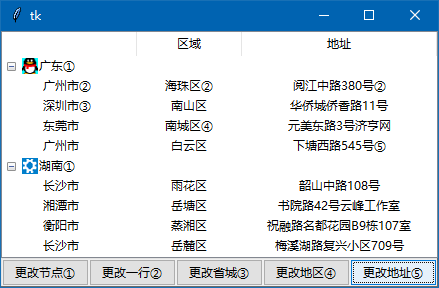### 修改标题栏

```tree1.heading('#0',text='改分类')

### 修改列宽，行高

`tree1.column('#01',width=150)`

`Style().configure('Treeview',rowheight=50)`

### 设置修改字体：

```style = Style()
style.configure("Treeview", font=(None, 20)) # 设置每一行的字体```

### Treeview组件的tags知识：

insert(父节点，插入位置，text=某值，values=某元组，tags=某字符串)

item( iid，tags=某字符串)    #  iid为某行的标识符

tag_configure( tags=某字符串， font=某值，background=某值…….)

```from tkinter import *
from tkinter.ttk import *

root = Tk()

tree1 = Treeview(root, columns=('qy', 'dz'))
# 创建树表格组件，栏目有3个：#0, qy, dz

tree1.column('#0', width=90, anchor=CENTER)
tree1.column('qy', width=90, anchor=CENTER)
tree1.column('dz', width=160, anchor=W)
# 定义3个栏目的宽度，对齐方法，宽度是否窗体变化

# 定义3个栏目的表头文字

tree1.insert('', END, text='广州市',values=('海珠区','阅江中路380号'))
tree1.insert('', END, text='深圳市',values=('南山区','华侨城侨香路11号'))
tree1.insert('', END, text='东莞市',values=('南城区','元美东路3号济亨网'))

tree1.insert('', END, text='长沙市',values=('雨花区','韶山中路108号'))
tree1.insert('', END, text='湘潭市',values=('岳塘区','书院路42号云峰工作室'))
tree1.insert('', END, text='衡阳市',values=('蒸湘区','祝融路名都花园B9栋107室'))

tree1.insert('', END, text='长沙市',values=('岳麓区','梅溪湖路复兴小区709号'))
tree1.insert('', END, text='广州市',values=('白云区','下塘西路545号'))

tree1.pack(fill=BOTH,expand=True)

def selectjob(event):
A=tree1.selection() # 获取当前选中行的iid
items = tree1.get_children()  # 获取根节点下所有行的iid，元组
print(items) # 大家可以看看这个iid元组是什么东西
for i in items: # 用循环把所有行再重新设置新的tags
tree1.item(i,tags='NONO')  # 重新设置tags

tree1.item(A,tags='OKOK') # 然后，再单独为当前选中行设置一个tags：OKOK
tree1.tag_configure('OKOK',font='黑体 12')  # 为tags(okok)设置另一字体及大小

tree1.update()  # 源码来自wb98.com

tree1.bind("<<TreeviewSelect>>",selectjob)

root.mainloop()```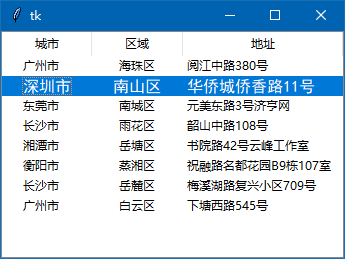### 隔行显示不同的颜色

下面我来做一个隔行显示不同颜色的代码，这个牵涉到背景颜色，我前面说，在3.8版的tkinter，要加多一些代码，才能让背景颜色起作用。

```def fixed_map(option):
return[elm for elm in style.map('Treeview',query_opt=option)
if elm[:2]!=('!disabled','!selected')]

style=Style()
style.map('Treeview',foreground=fixed_map('foreground'),
background=fixed_map('background'),)```

```from tkinter import *
from tkinter.ttk import *

def tree_color(): # 表格栏隔行显示不同颜色函数
items=tree1.get_children() # 得到根目录所有行的iid
i=0 # 初值
for hiid in items:
if i/2!=int(i/2): # 判断奇偶
tag1='' # 奇数行
else:
tag1='even' # 偶数行
tree1.item(hiid,tag=tag1) # 偶数行设为浅蓝色的tag='even'
i+=1 # 累加1

root = Tk() # 源码来自wb98.com

img1=PhotoImage(file='16-1.png')
img2=PhotoImage(file='16-2.png')

tree1 = Treeview(root, columns=('qy', 'dz'))
# 创建树表格组件，栏目有3个：#0, qy, dz

tree1.column('#0', width=120, anchor=CENTER)
tree1.column('qy', width=90, anchor=CENTER)
tree1.column('dz', width=180, anchor=CENTER)
# 定义3个栏目的宽度，对齐方法，宽度是否窗体变化

# 定义3个栏目的表头文字

tree1.insert('', END, text='广州市',values=('海珠区','阅江中路380号'))
tree1.insert('', END, text='深圳市',values=('南山区','华侨城侨香路11号'))
tree1.insert('', END, text='东莞市',values=('南城区','元美东路3号济亨网'))

tree1.insert('', END, text='长沙市',values=('雨花区','韶山中路108号'))
tree1.insert('', END, text='湘潭市',values=('岳塘区','书院路42号云峰工作室'))
tree1.insert('', END, text='衡阳市',values=('蒸湘区','祝融路名都花园B9栋107室'))

tree1.insert('', END, text='长沙市',values=('岳麓区','梅溪湖路复兴小区709号'))
tree1.insert('', END, text='广州市',values=('白云区','下塘西路545号'))

tree1.pack(fill=BOTH,expand=True)

# 3.8版要多加的代码------------
def fixed_map(option):
return [elm for elm in style.map("Treeview", query_opt=option)
if elm[:2] != ("!disabled", "!selected")]

style = Style()
style.map("Treeview", foreground=fixed_map("foreground"),
background=fixed_map("background"))
# 3.8版要多加的代码------------

#定义背景色风格
tree1.tag_configure('even', background='lightblue') # even标签设定为浅蓝色背景颜色

tree_color() # 启动程序，根据奇偶行设为不同的背景颜色

root.mainloop()```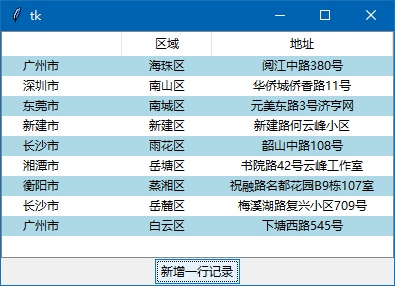### 一行记录的隐藏，重现，删除

delete(iid)  # 彻底删除一行，不能恢复

detach(iid)  # 假删除，只是隐藏

move(iid, parent,index) # 根据保存的iid, parent,index重新显示假删除的行

parent(item)：

index(item)

selection(items=None)

```from tkinter import *
from tkinter.ttk import *

def tree_color(): # 表格栏隔行显示不同颜色函数
items=tree1.get_children() # 得到根目录所有行的iid
i=0 # 初值
for hiid in items:
if i/2!=int(i/2): # 判断奇偶
tag1='' # 奇数行
else:
tag1='even' # 偶数行
tree1.item(hiid,tag=tag1) # 偶数行设为浅蓝色的tag='even'
i+=1 # 累加1

root = Tk()

img1=PhotoImage(file='16-1.png')
img2=PhotoImage(file='16-2.png')

tree1 = Treeview(root, columns=('qy', 'dz'))
# 创建树表格组件，栏目有3个：#0, qy, dz

tree1.column('#0', width=120, anchor=CENTER)
tree1.column('qy', width=90, anchor=CENTER)
tree1.column('dz', width=180, anchor=CENTER)
# 定义3个栏目的宽度，对齐方法，宽度是否窗体变化

# 定义3个栏目的表头文字

tree1.insert('', END, text='广州市',values=('海珠区','阅江中路380号'))
tree1.insert('', END, text='深圳市',values=('南山区','华侨城侨香路11号'))
tree1.insert('', END, text='东莞市',values=('南城区','元美东路3号济亨网'))

tree1.insert('', END, text='长沙市',values=('雨花区','韶山中路108号'))
tree1.insert('', END, text='湘潭市',values=('岳塘区','书院路42号云峰工作室'))
tree1.insert('', END, text='衡阳市',values=('蒸湘区','祝融路名都花园B9栋107室'))

tree1.insert('', END, text='长沙市',values=('岳麓区','梅溪湖路复兴小区709号'))
tree1.insert('', END, text='广州市',values=('白云区','下塘西路545号'))

tree1.pack(fill=BOTH,expand=True)

# 3.8版要多加的代码------------
# 源码来自wb98.com
def fixed_map(option):
return [elm for elm in style.map("Treeview", query_opt=option)
if elm[:2] != ("!disabled", "!selected")]

style = Style()
style.map("Treeview", foreground=fixed_map("foreground"),
background=fixed_map("background"))
# 3.8版要多加的代码------------

#定义背景色风格
tree1.tag_configure('even', background='lightblue') # even标签设定为浅蓝色背景颜色

tree_color() # 启动程序，根据奇偶行设为不行的背景颜色

tree1.selection_set('I001') # 默认选中第一项

fr1=Frame(root) # 收入3个按钮

def deljob(): # 真删除
iid=tree1.selection()
tree1.delete(iid)
tree_color() # 启动程序，根据奇偶行设为不行的背景颜色
def hidejob(): # 假删除，只是隐藏
global iid1,index1,parent1 # 定义为全局变量
iid1=tree1.selection() # 假删除前，记下选中前的iid
index1=tree1.index(iid1) # 记下假删除行的位置index
parent1=tree1.parent(iid1) # 记下假删除行的父节点
tree1.detach(iid1) # 假删除，隐藏
tree_color() # 删除及恢复记录后，根据奇偶行重新设置不同行的背景颜色

def showjob(): # 把假删除的记录在原来的位置显示
global iid1,index1,parent1 # 定义为全局变量
tree1.selection_set() # 取消以前的可能选中项
tree1.move(iid1,parent1,index1) # 在原来的位置恢复假删除的记录
tree_color() # 删除及恢复记录后，根据奇偶行重新设置不同行的背景颜色

but1=Button(fr1,text="隐藏",command=hidejob)
but1.pack(side=LEFT)
but1=Button(fr1,text="重现",command=showjob)
but1.pack(side=LEFT)
but1=Button(fr1,text="彻底删除",command=deljob)

root.mainloop()```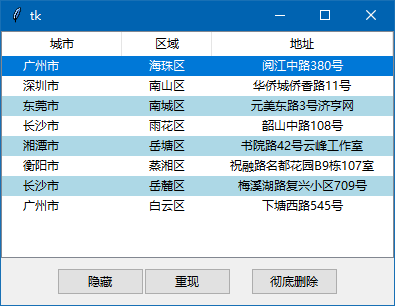```tree1.heading('#0', text='城市',command=lambda:print('城市栏'))

### 为不同的tag绑定不同的事件和函数

跟Text组件一样，我们可以为不同的tag标签绑定不同的事件和回调函数，方法也是类似的，语法：

tag_bind( tag, 事件，回调函数)

```tree1.tag_bind('1hang','<Button-1>',lambda even:print('奇数行')) # 1hang标签行绑定鼠标单击事件
tree1.tag_bind('2hang','<Button-1>',lambda even:print('偶数行')) # 2hang标签行绑定鼠标单击事件```

### Treeview组件加上水平和垂直滚动条

```from tkinter import *
from tkinter.ttk import *

root = Tk() # 源码来自wb98.com
root.geometry('300x200+888+444')

scr1=Scrollbar(root)  # 垂直滚动条
scr1.pack(fill=Y,side=RIGHT)
scr2=Scrollbar(orient=HORIZONTAL) # 水平滚动条
scr2.pack(fill=X,side=BOTTOM)

img1=PhotoImage(file='16-1.png')
img2=PhotoImage(file='16-2.png')

tree1 = Treeview(root, columns=('qy', 'dz'))
# 创建树表格组件，栏目有3个：#0, qy, dz

tree1.column('#0', width=120, anchor=CENTER)
tree1.column('qy', width=90, anchor=CENTER)
tree1.column('dz', width=180, anchor=CENTER)
# 定义3个栏目的宽度，对齐方法，宽度是否窗体变化

# 定义3个栏目的表头文字

sf1 = tree1.insert('', END, text='广东',image=img1,open=True)
sf2 = tree1.insert('', END, text='湖南',image=img2,open=True)
# 在根节点‘’下添加2个子节点：广东，湖南

tree1.insert(sf1, END, text='广州市',values=('海珠区','阅江中路380号'))
tree1.insert(sf1, END, text='深圳市',values=('南山区','华侨城侨香路11号'))
tree1.insert(sf1, END, text='东莞市',values=('南城区','元美东路3号济亨网'))
# 在广州（sf1）节点下，插入3条记录：#0栏 = text,其它栏 = values()

tree1.insert(sf2, END, text='长沙市',values=('雨花区','韶山中路108号'))
tree1.insert(sf2, END, text='湘潭市',values=('岳塘区','书院路42号云峰工作室'))
tree1.insert(sf2, END, text='衡阳市',values=('蒸湘区','祝融路名都花园B9栋107室'))
# 在湖南（sf2）节点下，插入3条记录：#0栏 = text,其它栏 = values()

tree1.insert(sf2, END, text='长沙市',values=('岳麓区','梅溪湖路复兴小区709号'))
tree1.insert(sf1, END, text='广州市',values=('白云区','下塘西路545号'))
# 以同样方法插入2条记录，它们会根据父节点找到自己的位置

tree1.pack(fill=BOTH,expand=True)

# 以下绑定水平和垂直滚动条
tree1.config(xscrollcommand = scr2.set)
scr2.config(command =tree1.xview)

tree1.config(yscrollcommand = scr1.set)
scr1.config(command = tree1.yview)

root.mainloop()```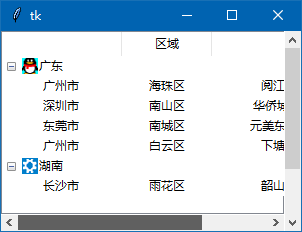Treeview 组件要学的知识太多了，Treeview组件知识我暂时讲到这，还有些知识，我以后有机会再讲。

<< 上一篇 下一篇 >>